Click to Chat

1800-1023-196

+91-120-4616500

CART 0

• 0

MY CART (5)

Use Coupon: CART20 and get 20% off on all online Study Material

ITEM
DETAILS
MRP
DISCOUNT
FINAL PRICE
Total Price: Rs.

There are no items in this cart.
Continue ShoppingSolved Examples on Equilibrium

Question 1:Calculate  the pH of the solution when 0.1 M CH3 COOH (50 ml) and 01. M NaOH (50 ml) are mixed, [Ka (CH3COOH)=10-5]

Solution:

CH3 COOH   CH3 COO_ + H+ …(I)

NaOH →  Na+ + OH-

H+ + OH_ H2O …(II)

(I) + (II)

CH3COOH + OH-   CH3COO- + H2O . (III)

0.05-X         0.05-x         x

Keq of eq. (III) = Ka/Kw

conc. of H2O remain constant

109 = x/(0.05-x)2

because value of eq. Const.is very high

here for x» 0.05

let 0.05-x=a

109=0.05/a2

a = 7.0710-6

pOH= 6-log 7.07

pOH= 6 – 0.85

pH= 14-6+0.85 = 8.85

________________________________________________________________________________________

Question 2:

Calculate the pH at the equivalence point of the titration between 0.1M CH3­COOH ( 25 ml) with 0.05 M NaOH. Ka (CH3COOH) = 1.8  10–5.

Solution:

We have already seen that even though when CH3COOH is titrated with NaOH the reaction does not go to completion but instead  reaches equilibrium. We can assume that the reaction is complete and then salt gets hydrolysed because, this assumption will help us to do the problem easily and it does not effect our answer.

First of all we would calculate the concentration of the salt, CH3COONa.  For reaching equivalence point,

N1V1 = N2V2

0.1 ´ 25 = 0.05 ´ V2

V2 = 50 ml

Therefore [CH3COONa] = (0.125)/75 =0.1/3

[H+] =

[H+] = 2.32 ´ 10–5

pH = – log 2.32 ´ 10–5 = 8.63

___________________________________________________________________________

Question 3:

Given the solubility product of Pb3 (PO4)2 is 1.5 x 10-32.Determine the solubility in gms/litre.

Solution:

Solubility product of Pb3 (PO4)2 = 1.5  10–32

Pb3 (PO4)2  3Pb2+ + 2PO43-

If x is the solubility of Pb3 (PO4)2

Then Ksp = (3x)3 (2x)2 = 108 x5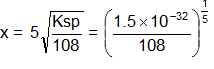x = 1.692  10–7 moles/lit

Molecular mass of Pb3(PO4)2 = 811

x = 1.692 ´ 10–7 ´ 811 g/lit = 1.37  10–4 g/lit

Solubility product is

Ksp(SrC2O4) = [Sr2+] [C2O42–] = (5.4  10–4)2-    = 2.92  10–7

________________________________________________________________________________________

Question 4:

What is pH of 1M CH3COOH solution? To what volume must one litre of this solution be diluted so that the pH of resulting solution will be twice the original value. Given : Ka = 1.8  10–5

Solution:

H3CCOOH      +    H2O          H3CCOO– +    H3O+

t = 0                 1M                         0                0

-xM                  xM                         xM

__________________________________________

t = teq               (1-x)M                   x                xpH = – log [H3O+] = – log {4.2  10–3} = 3 – log 4.2  = 2.37

Now, let 1L of 1M ACOH solution be diluted to VL to double the pH and the conc. of diluted solution be C.

H3CCOOH + H2O      H3CCOO–      +       H3O+

t = 0          C                                 0                           0

– 1.8  10–5                   1.8  10–5               1.8  10–5

____________________________________________________

t= teq     C – 1.8  10–5             1.8  10–5              1.8  10–5

New pH = 2  old pH = 2  2.37 = 4.74

pH = – log [H3O+] = 4.74

[H3O+] = 1.8  10–5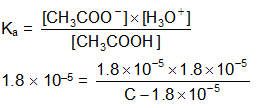C = 3.6  10–5 L

on dilution

M1V1 = M2V2

1M  1L = 3.6  10–5 L  V2

V­2 = 2.78  104 L

________________________________________________________________________________

Question 5 :

Find the concentration of H+, HCO3- and CO32-, in  a0.01M solution of carbonic acid if the pH of this is 4.18.

Ka1(H2CO3) = 4.45  10–7 and Ka2 = 4.69  10–11

Solution:

pH = – log[H+]

4.18 = – log [H+]

[H+] = 6.61  10–5

H2CO3  H+ + HCO3-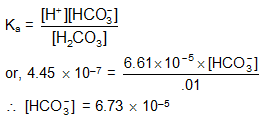again, HCO3-  H+ + CO32-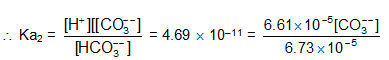[CO32-] = 4.8  10–11

______________________________________________________________________________________

Question 6:

Calculate the molar solubility of Mg(OH)2 in 1MNH4Cl

KspMg(OH)2 = 1.8  10–11

Kb(NH3) = 1.8  10–5

Solution:

Mg(OH)2(s)  Mg++ + 2OH–         K1 = Ksp

2NH4+ + 2OH-  2NH4OH          K2 = 1/K2b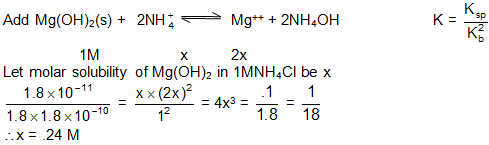____________________________________________________________________________________

Question 7:

An aqueous solution of metal bromide MBr2 (0.05M) in saturated with H2S. What is the minimum  pH at which MS will ppt.?

Ksp =(MS) =  6  10–21

Concentration of standard H2S = 0.1

Ka1(H2S) = 1  10–7

Ka2(H2S) = 1.3  10–13

Solution:

In saturated solution of MS

MS(s)   M++ + S2-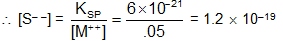The precipitate of MS will form only if [S––] exceeds the concentration of 1.2  10–19

H2S  H+ + HS–           Ka1

H2S–   H+ + S--           Ka2

——————————————

H2S   2H+ + S2–         K = 1.3  10–20[H+] = 0.109

pH = 0.96

___________________________________________________________________________________

Question 8 :

How much AgBr could dissolve in 1.0 L of 0.4 M NH3? Assume that [Ag (NH3)2]+  is the only complex formed given, Kf [Ag(NH3)2+]=1.0108, Ksp (AgBr)= 5.010-13

Solution:

AgBr  Ag+ + Br-

Ag+ + 2NH3  Ag (NH2)2+

Let x= solubility ,

Then x= [Br-]=[Ag+]+[Ag(NH3)2+]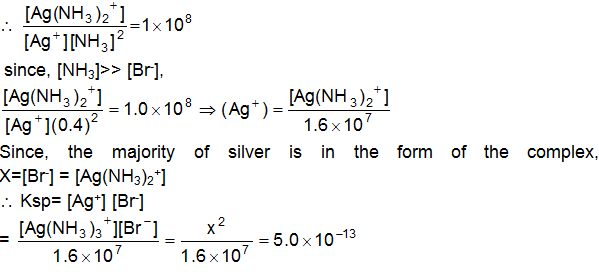x2=8.0´10-6

x=2.8´10-3 M

_______________________________________________________________________________________________

Question 9:

Equal volumes of 0.02 M Ag NO3 and 0.02 M HCN were mixed. Calculate [Ag+] at equilibrium given, Ksp (AgCN)= 2.210-16

Ka (HCN)= 6.210-10

Solution.

Initially, assume complete precipitation

Ag+ + HCN →  AgCN + H+, since the solution

were diluted to double volume

concentration of [H+] = 0.02/2 = 0.01M

Now consider the equilibrium

AgCN  Ag+ + CN-      Ksp = 2.2´10-16= [Ag+] [CN-]

HCN  H+ + CN-          Ksp = 6.2´10-10= [H+] [CN-]/[HCN]

Since every dissolved CN- is also hydrolyzes into HCN up to certain extent.__________________________________________________________________________________________________

Question 10 :

What is solubility of PbS (a) ignoring the hydrolysis of ions (b) including the hydrolysis of ions (assume pH of solution  = 7).

Given that:Solution:

a)  Pbs(S)   Pb++ + S––

Ksp = [Pb++] [S––] = S ´ S = S2 = 7 ´ 10–29

S = 8.4  10–15

b)  Including hydrolysis: The equilibria of interest areMass balance expression are:

[Pb2+]o = [Pb2+] + [Pb(OH)]+ ------------- (a)

[S--]o = [S––] + [HS–] + [H2S] ------- (b)

Substituting the value of [Pb(OH)+] from equation (i) into equation (a)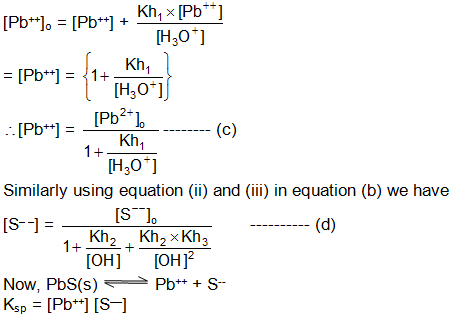Substituting the values of [Pb++] and [S––] from equations (c) and (d), we get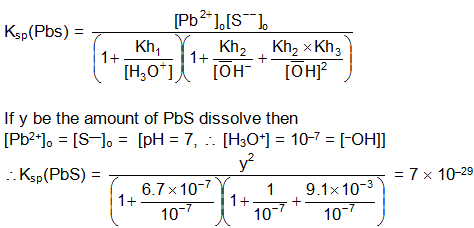On solving, Y = 1.0146  10–10

______________________________________________________________________________________

Question 11:

Calculate the solubility of MnS in pure water. Assume hydrolysis of S2– ions.

Ksp(MnS) = 2.5  10–10

Ka1 and Ka2 of H2S are 1 ´ 10–7 and 1  10–14 respectively

Solution:

Let molar solubility of MnS be XM

Mn(s)  Mn2+ + S2-As K’h >>K”h, first step hydrolysis is almost complete,

x  = [Mn2+] = [HS–] = [OH–]

Consider first step hydrolysis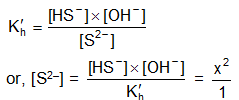At equilibrium, [Mn2+] [S2–] = Ksp = 2.5  10–10

Or n = (2.510-10)/X2

x = 6.3 10–4 M

_____________________________________________________________________________________________________________________

Question 12:

How much solid Na2S2O3 should be added to 1.0 L of water so that 0.0005 mole Cd (OH2) could just barely dissolve ?

K1 and K2 for S2O32- complexation with Cd2+ are 8.3103 and 2.5102, respectively. Ksp (Cd(OH)2= 4.510-15

Solution:

Cd(OH)2  Cd2+ + 2OH-

Ksp = [Cd2+] [OH-]2 =4.5´10-15

Cd2+ + S2O32- Cd (S2O3)

Cd (S2O3) + S2O3--  Cd (S2O3)2--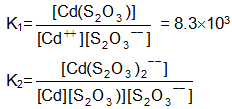K2=2.5102

Assume that S2O3-- dose not hydrolyze

[Cd2+] + [Cd(S2O2)] + [Cd(S2O3)22-] = 0.00050

[Cd2+]+K1 [Cd ++] S2O3--] + K1K2 [Cd2+] [S2O32-]2= 0.00050

[Cd2+]= Ksp/[OH-]2 = 4.510-9 M

let [S2O32--]=x

then, 1+K1x+K1K2x2= 1.1  105

x = 0.2009

Wt/Mwt = 0.2009

wt = 0.2009 158 =31.74

_____________________________________________________________________________________________________

Question  13:

A solution contains a mixture of Ag+ (0.1M) and Hg22+ (0.1M) which are to be separated by selective precipitation. Calculate the maximum concentration of iodide ion at which one of them gets precipitated almost completely. What percentage of that metal ion is precipitated?

Ksp (AgI) = 8.5  10–17

Ksp (Hg2I2) = 2.5  10–26

Solution:

Let us first calculate [I–] to precipitate AgI and Hg2I2

Ksp[AgI] = [Ag+] [I–]

8.5  10–17 = (0.1) [I–]

[I–] to precipitate as AgI = (8.5 10-17)

Ksp(Hg22+) = [Hg2I2][I–] = 8.5  10–16 M

2.5  10–26 = 0.1 [I–]2

[I–] to precipitate Hg2I2 = 5.0  10–13 M

[I–] to precipitate AgI is smaller. Therefore, Ag I will start precipitating first. On further addition of I– more AgI will precipitate and when [I–] ³ 5.0 ´ 10–13 J, Mg2I2 will start precipitating. The maximum concentration of Ag+ at this stage will thus be calculated as:

Ksp(AgI) = [Ag+] [I–]

8.5  10–17 = [Ag+] (5.0 ´ 10–13)

or, [Ag+] = 1.7  10–4 M

Percentage of Ag + remained precipitated = [(1.7  10–4 M)/0/1]100 = 0.17%

Thus percentage of Ag+ precipitated = 99.83%

______________________________________________________________________________________________________

Question 14:

What [H+] must be maintained in a saturated H2S (0.1M) to precipitate CdS but not ZnS, if [Cd+2] = [Zn+2] = 0.1 (M) initially?

Solution:

In order to prevent precipitating of ZnS,

[Zn+2] [S–2] < Ksp (ZnS) = 1  10–21

Ionic product

or, 0.1 [S–2] < 1  10–21

or, [S–2] < 1  10–20

This is the maximum value of [S–2] before ZnS will precipitate

Let is the maximum value of [S–2] be x.

Thus for H2S  2H+ + S–2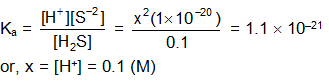or, x = [H+] = 0.1 (M)

No ZnS will precipitate at and concentration of H+ greater than 0.1M.

_______________________________________________________________________________________________

Question 15:

Assuming the complete dissociation of HCl and the lead salt, calculate how much HCl is added to 0.001M lead salt solution to just percent precipitation when saturated with H2S. The concentration of H2S in its saturated solution is 0.1M

Ka (H2S) = 1.1  10–23

Ksp (PbS) = 3.4  10–28

Solution:

We know, Ksp(PbS) = [Pb+2] [S–2]

Since lead salt is completely dissociated, [Pb+2] is equal to the concentration of lead salt, i.e. [Pb+2] = 0.001M. If[S–2] is the concentration of S–2 required to just start precipitation of PbS.

[S–2]  = 3.4  10–25

Now the addition of HCl with suppress the dissociation of H2S to that extent that [S–2] = 34 ´ 10–25 (M)

HCl is completely ionised, \ [H+] = [HCl]

Let [HCl] be x’. Therefore [H+] = x’

H2S  2H+ + S–2

At equilibrium

[H2S] = 0.1 – 3.4  10–25 ≈ 0.1

[H+] = 2  3.4  10–25 + x’ ≈ x’

[S–2] = 3.4  10–25

Related Resources:-

Look into the Sample Papers of Previous Years

You can get the knowledge of Useful Books of Chemistry

To read more, Buy study materials of Chemical Equilibrium comprising study notes, revision notes, video lectures, previous year solved questions etc. Also browse for more study materials on Chemistry here.### Course Features

• 731 Video Lectures
• Revision Notes
• Previous Year Papers
• Mind Map
• Study Planner
• NCERT Solutions
• Discussion Forum
• Test paper with Video Solution